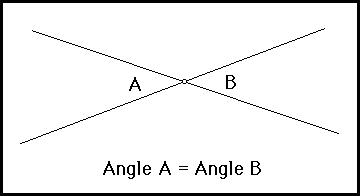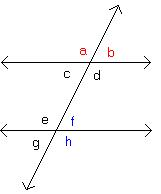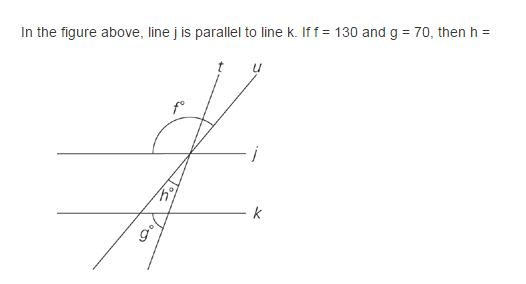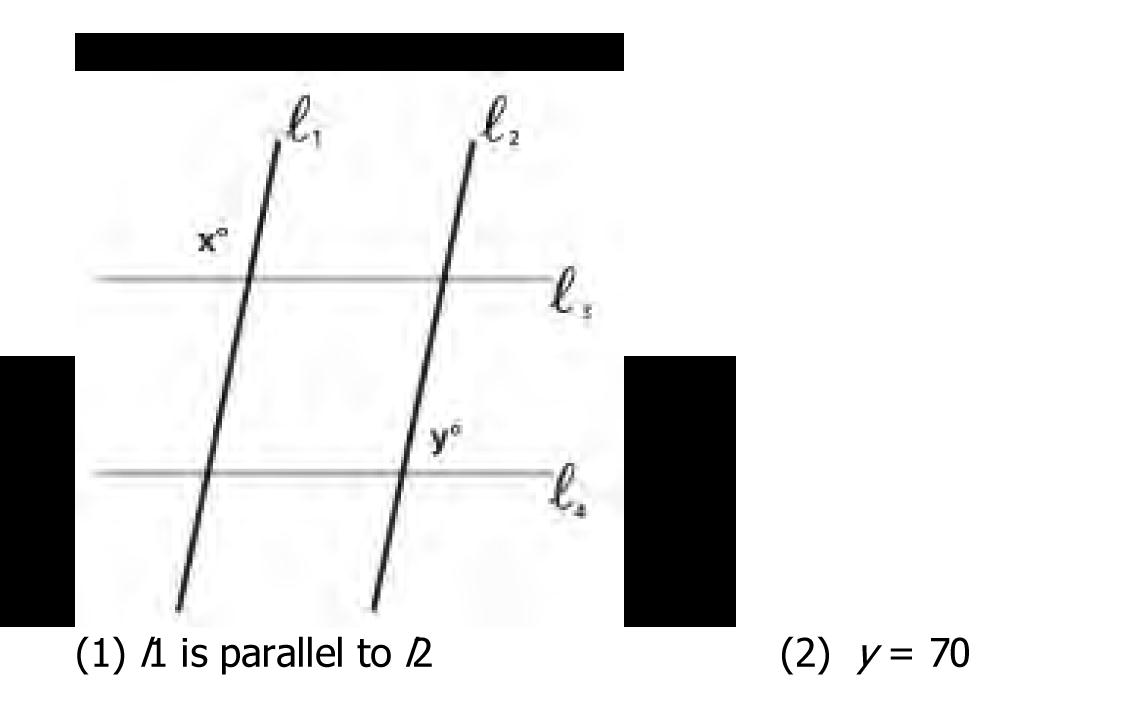Option Training Institute FZ LLC G 37 & G 34, Block 13, Al Sufouh Road Knowledge, Village Dubai PO Box : 501703

# GEOMETRY: AS ON THE GMATGMAT geometry is always very logical. Most of the time GMAT gives you a figure and some information to solve the missing angles or sides of that figure. Geometry in Problem Solving is different from geometry in Data sufficiency type as in most of the questions in PS, the figure is always drawn to scale unless stated by the question.

The very first concept in Geometry is Lines and angles. There are only three properties to solve all lines and angles questions.

1. Intersecting lines/vertical angles

When two lines intersect, the angles created by these lines possess special qualities. The opposite angles are equal in measure. These opposite angles are also known as vertical angles. The sum of adjacent angles equals 180 degrees.Angle A and Angle B are Vertical Angles.

2. If two lines are not intersecting the lines are parallel. When two parallel lines are intersected by a third, then two intersections and vertical angles are created.Angle a = Angle d = Angle e = Angle h

Angle g = Angle f = Angle c = Angle b

3. Sum of Interior Angles = (n-2) × 180°

Each Angle (of a Regular Polygon) = (n-2) × 180° / nExplanation:

If f = 130, then both large angles formed by lines t and k also measure 130°. The small angles formed by those two lines therefore measure 50° (notice that one of these angles is the left base angle of the triangle). If g = 70, then the angle above it (the other base angle of the triangle) must measure 110° to complete the 180° in a straight line. So far, you have 160° in the triangle. To complete the 180° total in the triangle, h must measure 20°.

Data Sufficiency Question:Explanation:

(1) INSUFFICIENT: We don’t know any of the angle measurements.

(2) INSUFFICIENT: We don’t know the relationship of x to y.

(1) AND (2) INSUFFICIENT: Because l1 is parallel to l2, we know the relationship of the four angles at the intersection of l2 and l3 (l3 is a transversal cutting two parallel lines) and the same four angles at the intersection of l1 and l3. We do not, however, know the relationship of y to those angles because we do not know if l3 is parallel to l4.